## 一、面向对象的基本概念

### 1.类和对象

``````class 类名{
类的描述
}
``````

``````\$obj = new 类名();
``````

``````<?php
class Dog{
var \$color = "";
function speak(){
echo "wangwang";
}
}

\$dog1 = new Dog();

\$dog1 -> color = "red";

\$dog1 -> speak();
``````

### 3.对象的创建形式：

1.方法一：

``````\$obj = new 类名();
``````

2.方法二：

``````\$a = "类名"；

\$obj = \$a();
``````

3. 方法三:

``````\$obj = new 类名();
\$obj1 = new \$obj();
``````

4.方法四：

``````\$obj = new self;
``````

### 5.对象的值传递

``````<?php

class Dog{
var \$p1 =1;
}

\$t1 = new Dog();
\$t2 = \$t1;

echo "t1中的p1 ".\$t1->p1."<br>";
echo "t2中的p1 ".\$t2->p1."<br>";

\$t1->p1 = 11;

echo "赋值后t1中的p1 ".\$t1->p1."<br>";
echo "赋值后t2中的p1 ".\$t2->p1."<br>";
``````

``````t1中的p1 1
t2中的p1 1

``````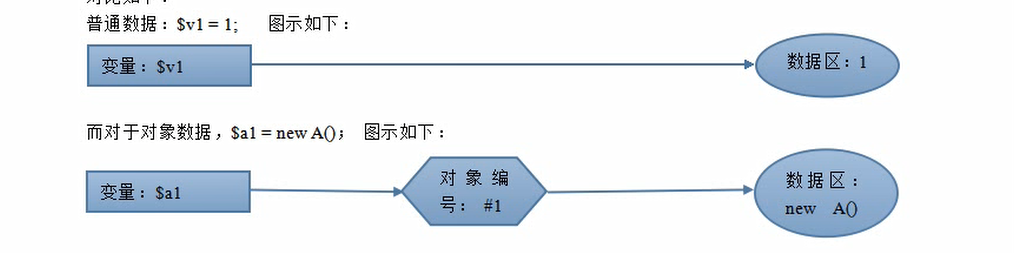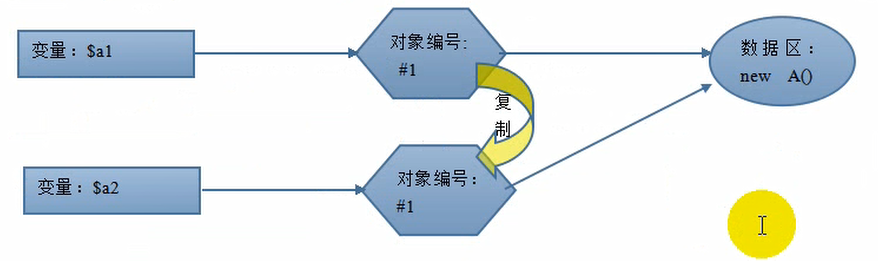``````<?php

class Dog{
var \$p1 =1;
}

\$t1 = new Dog();
\$t2 = \$t1;

echo "t1中的p1 ".\$t1->p1."<br>";
echo "t2中的p1 ".\$t2->p1."<br>";

\$t1->p1 = 11;

echo "赋值后t1中的p1 ".\$t1->p1."<br>";
echo "赋值后t2中的p1 ".\$t2->p1."<br>";

\$t2 = &\$t1;
\$t1->p1 = 111;

echo "赋值后t1中的p1 ".\$t1->p1."<br>";
echo "赋值后t2中的p1 ".\$t2->p1."<br>";
``````

``````t1中的p1 1
t2中的p1 1

``````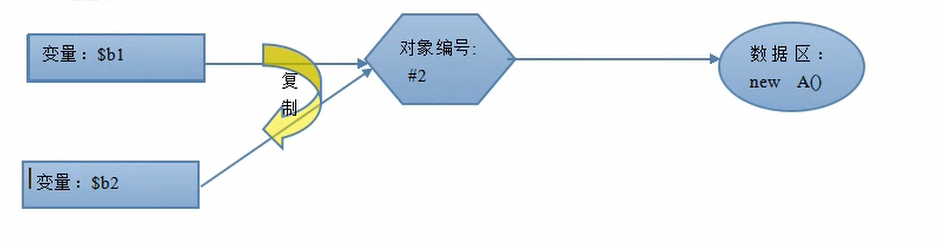### 6.静态属性和静态方法

#### 定义格式：

static 属性名[=xxx]；
static function 方法名(){xxx};

#### 访问格式：

``````类名：：\$属性名

``````

``````<?php

class Dog{
static \$p1 =1;
static function hello(){
return "helo world";
}
}

echo Dog :: hello()."<br>";
echo Dog :: \$p1;
``````

``````helo world
1
``````

### 7.this 和 self 关键字

\$this 代表当前类的对象
self 代表类本身

self 在类内部改变静态变量的值很有用
\$this 不能用在静态方法中，因为调用静态方法的是类而不是类对象

### 8.类常量

``````class 类名{
const 常量名 = 常量值；
}
``````

### 10.析构方法

#### 2.那么什么时候对象会被销毁呢？

(1)当网页代码执行结束的时候，所有的对象都会被销毁，（变量会按照生成的顺序逆序的销毁）
(2)当一个对象没有变量引用的时候（引用计数机制）

## 二、类的继承

### 1.基本概念：

``````<?php

class A{
function speak(){
echo "wangwang"."<br>";
}
}

class B extends A{
function move(){
echo "i can move<br>";
}
}

\$dog = new B();
\$dog->speak();
\$dog->move();
``````

``````wangwang
i can move
``````

### 3.单继承

PHP 和大多数的面向对象的语言都是单继承模式，C++ 支持多继承， Python 也支持多继承

### 4.访问控制修饰符

public : 谁都能访问，var 在修饰属性的时候和 public 是一样的
protected ： 只有自己家族内部可以访问（具有继承关系的多个类之间）
private ： 只有自己可以访问

``````<?php

class Person{
public \$name;
protected \$age;

function __construct(\$name,\$age,\$pass){
\$this->name = \$name;
\$this->age = \$age;
}

}

\$xiaoming = new Person("小明",18,"123456");
echo \$xiaoming->name;
echo \$xiaoming->age;
``````

``````<?php

class Person{
public \$name;
protected \$age;

function __construct(\$name,\$age,\$pass){
\$this->name = \$name;
\$this->age = \$age;
}

function showInfo(){
echo \$this->name."<br>";
echo \$this->age."<br>";
}

}

\$xiaoming = new Person("小明",18,"123456");
\$xiaoming->showInfo();
``````

``````小明
18
123456
``````

### 5.构造方法和析构方法在继承中的表现

``````<?php

class A{
function __construct(){
echo "父类A的构造方法<br>";
}
}

class B extends A{
function __construct(){
echo "子类B的构造方法<br>";
parent :: __construct();
}
}

\$b = new B();
``````

``````子类B的构造方法

``````

### 6.parent 关键字

parent 关键字用来表示某一个类的父类（或者说父类的对象），类似于Python 中的 super()，常常在子类中去访问父类的方法或者属性，他的使用形式只有一个

``````parent :: 父类的属性或者方法
``````

``````<?php

class Person{
public \$name = " ";
public \$age = " ";
function __construct(\$name,\$age){
\$this -> name = \$name;
\$this -> age = \$age;
}
function showInfo(){
echo "xxxx";
echo "yyyy";
}
}

class Teacher extends Person{
public \$edu;
function __construct(\$name, \$age ,\$edu)
{
parent::__construct(\$name, \$age);
\$this -> edu = \$edu;
}

function showInfo()
{
parent::showInfo(); // TODO: Change the autogenerated stub
echo "zzzz";
}
}
``````

### 7.重写

#### 1.重写的要求

(1)子类重写上级类成员时，访问控制修饰符不能权限更低（意思是可以更开放）

(2) 子类重写父类的方法是要求形参保持一致

## 三、设计模式

### 2.工厂模式

``````<?php

class Factory{
static function getObject(\$class_name){
\$obj = new \$class_name();
return \$obj;
}
}
``````

### 3.单例模式

``````<?php

class Single{
static \$s = null;
function __construct(){
}
function getOne(){
if(empty(self::\$s)){
self::\$s = new self();
return self::\$s;
}
else{
self::\$s;
}
}
}
``````

## 四、抽象类和抽象方法

### 1.抽象类

#### 1.概念：

``````abstruct class 类名{
类成员
}
``````

### 2.抽象方法

abstruct function 方法名 (形参);

### 3.抽象类和抽象方法的关系

(1)一个类中有抽象方法那么这个类必须声明为抽象类
(2)一个类继承一个抽象类那么就必须实现所有的抽象方法，除非这个类也是作为一个抽象类
(3)子类实现父类的抽象方法的时候访问控制修饰符权限不能降低（可以更加开放），并且形参需要一致

### 1.概念

php 中的重载指的是，当我们使用对象（或者类）去访问一些不存在的属性或者方法的时候，就会使用某些预先定义好的特殊方法来应对这种情况

1.属性重载
2.方法重载

### 3.属性重载：

``````__set(\$name,\$value)

__get(\$name)

__isset(\$name)

__unset(\$name)
``````

#### 1.`__set(\$name,\$value)`

``````<?php

class A{
public \$p1 = 1;
public \$prop_list = array();
function __set(\$name, \$value)
{
// TODO: Implement __set() method.
\$this->prop_list[\$name] = \$value;
}
}

\$a = new A();
\$a->p1 = 11;
\$a->p2 = 22;
var_dump(\$a);
``````

``````object(A)
public 'p1' => int 11
public 'prop_list' =>
array (size=1)
'p2' => int 22
``````

#### 2.`__get(\$name)`

``````<?php

class A{
public \$p1 = 1;
public \$prop_list = array();
function __set(\$name, \$value)
{
// TODO: Implement __set() method.
\$this->prop_list[\$name] = \$value;
}

function __get(\$name)
{
// TODO: Implement __get() method.
if(!empty(\$this->prop_list[\$name])) {
return \$this->prop_list[\$name];
}else{
return "该属性不存在";
}
}

}

\$a = new A();
\$a->p1 = 11;
\$a->p2 = 22;
echo \$a->p2;
``````

``````22
``````

#### 3.`__isset(\$name)`

``````class A{
public \$p1 = 1;
public \$prop_list = array();
function __set(\$name, \$value)
{
// TODO: Implement __set() method.
\$this->prop_list[\$name] = \$value;
}

function __get(\$name)
{
// TODO: Implement __get() method.
if(!empty(\$this->prop_list[\$name])) {
return \$this->prop_list[\$name];
}else{
return "该属性不存在";
}
}

function __isset(\$name)
{
// TODO: Implement __isset() method.
if(isset(\$this->prop_list[\$name])){
return true;
}else{
return false;
}
}

}

\$a = new A();
\$a->p1 = 11;
\$a->p2 = 22;
echo isset(\$a->p2);
``````

``````1
``````

#### 4.`__unset(\$name)`

``````<?php

class A{
public \$p1 = 1;
public \$prop_list = array();
function __set(\$name, \$value)
{
// TODO: Implement __set() method.
\$this->prop_list[\$name] = \$value;
}

function __get(\$name)
{
// TODO: Implement __get() method.
if(!empty(\$this->prop_list[\$name])) {
return \$this->prop_list[\$name];
}else{
return "该属性不存在";
}
}

function __isset(\$name)
{
// TODO: Implement __isset() method.
if(isset(\$this->prop_list[\$name])){
return true;
}else{
return false;
}
}

function __unset(\$name)
{
// TODO: Implement __unset() method.
if(isset(\$this->prop_list[\$name])){
unset(\$this->prop_list[\$name]);
}else{
echo "error";
}

}

}

\$a = new A();
\$a->p1 = 11;
\$a->p2 = 22;
unset(\$a->p2);
``````

### 4.方法重载：

callstatic(\$name,\$arguments)

``````\$name 不存在的函数名

\$arguments 所有参数组成的数组
``````

#### 1.`__call(\$name,\$arguments)`

``````<?php

class A{
function __call(\$name, \$arguments)
{
// TODO: Implement __call() method.
if(\$name == "eat"){
\$num = count(\$arguments);
if(\$num ==1){
\$this->hezhou(\$arguments);
}
if(\$num ==2){
\$this->chifan(\$arguments,\$arguments);
}
}
}

function hezhou(\$zhou){
echo "zheng zai he \$zhou <br>";
}

function chifan(\$fan,\$tools){
echo "zheng zai yong \$tools chi \$fan <br>";
}
}

\$p = new A();
\$p->eat("zhou");
\$p->eat("fan","kuaizi");
``````

``````zheng zai he zhou
zheng zai yong kuaizi chi fan
``````

## 六、接口

### 1.概念：什么是接口

``````interface class A{
const PI = 3.14;
function B(); //接口中的方法都是抽象方法，不用写 abstract
}
``````

### 2.为什么需要接口

``````class 类名 implements 接口名{
成员定义
}
``````

``````<?php

interface Player{//音乐播放器接口
function play();
function next();
function prev();
function stop();
}

interface USBset{//USB接口
const WIDTH = 12;
const HEIGHT = 5;
function data_in();
function data_out();
}

class MP3Player implements Player,USBset{
function play(){}
function next(){}
function prev(){}
function stop(){}
function data_in(){}
function data_out(){}
}
``````

### 3.关于接口的其他细节

1.一个类在实现接口的同时也能继承父类
2.接口之间也可以相互继承
3.接口中的常量和抽象方法只能是 Public 无需写 abstruct

## 七、MVC模式

1.需求分析

2.项目概要和详细设计

3.界面设计

4.前端页面制作

5.前后台功能实现

6.功能测试

7.发布上线，运行维护

### 2.显示与逻辑相分离的思想

1.显示年月日
2.显示时分秒
3.两者都 显示

#### 1.显示与逻辑混合

``````<html>
<body>
<p align = right>
<a href="?type=1">形式一</a>
<a href="?type=2">形式一</a>
<a href="?type=3">形式一</a>
<hr>
</p>

<?php

if(!empty(\$_GET['type']) && \$_GET['type'] == "1"){
\$t1 = date("Y年m月d日");
}elseif(!empty(\$_GET['type']) && \$_GET['type'] == "2"){
\$t1 = date("H:i:s");
}else{
\$t1 = date("Y年m月d日 H:i:s");
}

echo "<h1>\$t1</h1>";
?>
</body>
</html>
``````

#### 2.显示与逻辑相分离

PHP 文件

``````show-time.php

<?php

if(!empty(\$_GET['type']) && \$_GET['type'] == "1"){
\$t1 = date("Y年m月d日");
}elseif(!empty(\$_GET['type']) && \$_GET['type'] == "2"){
\$t1 = date("H:i:s");
}else{
\$t1 = date("Y年m月d日 H:i:s");
}
include "./show-time.html";
?>
``````

HTML文件

``````show-time.html

<html>
<body>
<p align = right>
<a href="?type=1">形式一</a>
<a href="?type=2">形式一</a>
<a href="?type=3">形式一</a>
<hr>
</p>

<?php
echo "<h1>\$t1</h1>";
?>
</body>
</html>
``````

### 3.MVC框架原理

M: Model 模型
V: View 视图
C：Controller 控制器

#### 2.MVC 的简单演示

show-time-controller.php

``````<?php

require_once "show-time-model.php";

if(!empty(\$_GET['type']) && \$_GET['type'] == "1"){
\$obj = new GetTimeModel();
\$t1 = \$obj->GetDate();

}elseif(!empty(\$_GET['type']) && \$_GET['type'] == "2"){
\$obj = new GetTimeModel();
\$t1 = \$obj->GetTime();

}else{
\$obj = new GetTimeModel();
\$t1 = \$obj->GetDateTime();

}

include "./show-time-view.html";
?>
``````

show-time-model.php

``````<?php

class GetTimeModel{
function GetDate(){
return Date("Y年m月d日");
}

function GetTime(){
return  Date("H:i:s");
}

function GetDateTime(){
return Date("Y年m月d日 H:i:s");
}

}
``````

show-time-view.html

``````<html>
<body>
<p align = right>
<a href="?type=1">形式一</a>
<a href="?type=2">形式一</a>
<a href="?type=3">形式一</a>
<hr>
</p>

<?php
echo "<h1>\$t1</h1>";
?>
</body>
</html>
``````

#### 4.MVC思想框架

1.浏览器直接请求的是控制器文件，也只有控制器文件知道用户给了什么（请求数据）以及用户真正要的是什么（目标）

2.控制器根据用户的请求做两件事
（1）调用哪个模型
（2）获取什么数据
（3）显示数据到哪个视图中（其实就是载入视图文件）

3.模型文件：只根据控制器的调用生产数据并返回给控制器

4.视图文件：只负责显示数据（数据显示在页面的哪里以及显示的效果），并且是由控制器决定显示哪些数据

5.模型文件和视图文件没有直接的关系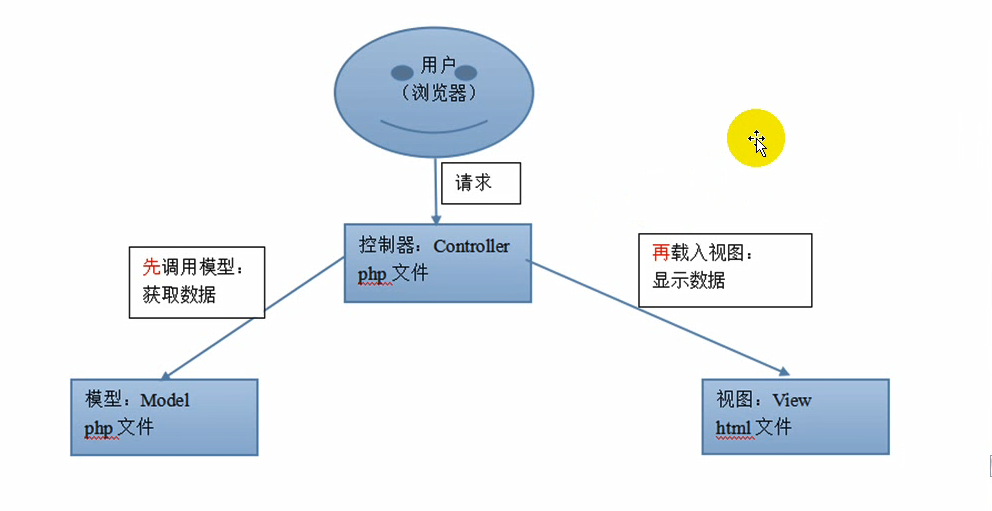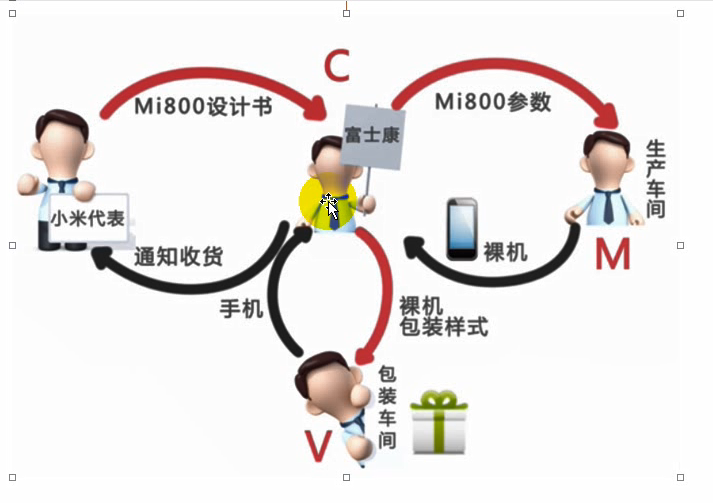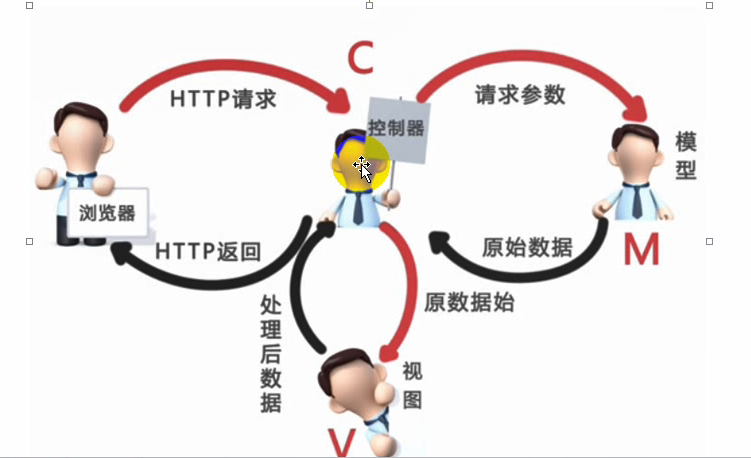### 4.Model 层的典型实现

#### 1.模型层的功能与典型的代码模式

``````class ModelDemo{
function f1(){
\$result = 获取数据库中的数据1；
return \$result;
}

function f2(){
\$result = 获取数据库中的数据2；
return \$result;
}

...
}
``````

#### 2.控制器层的功能与典型的代码模式

1.载入模型类文件
2.对模型类实例化以获得相应的对象
3.调用模型类对象的方法以获得相应的数据（不同的方法能获得不同的数据）

``````require_once "模型文件"

class xxxController{
function xxxAction(){
\$obj = new 模型类();
\$res1 = \$obj->方法1();

}

function yyyAction(){
\$obj = new 模型类();
\$res2 = \$obj->方法2();
}

...
}

\$controller = new xxxController();

\$action = !empty(\$_GET['action']) ? \$_GET['action'] : "Index";

\$action = \$action."Action";

\$controller->\$action();
``````

``````class xxxModel{
...
}
``````

``````xxxModel.class.php
``````

#### 3.模型类的单例工厂

ModelFactory.class.php

``````class ModelFactory{
static \$arry = array();
static function M(\$class_name){
if(empty(self::\$array[\$class_name])){
self::\$array[\$class_name] = new \$class_name();
}
return self::\$array[\$class_name];
}
}
``````

#### 4.整个模型层的类库结构图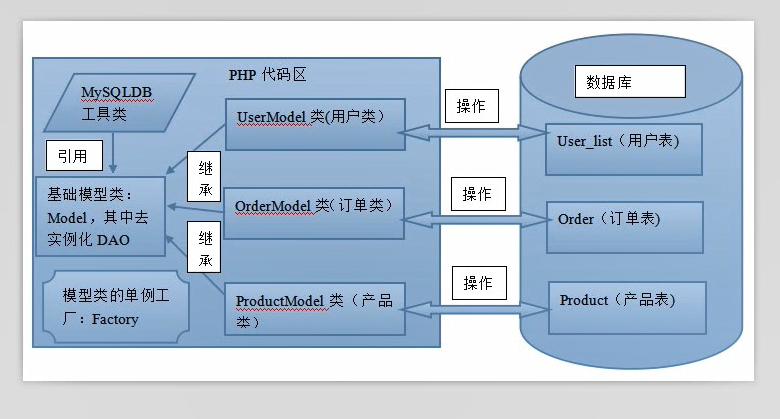1.功能

2.划分：

3.与模型的区别：

4.动作

### 6.View 层的典型实现

1.视图层的功能：

2.数据的分类：

(1)普通标量数据

``````<?php echo "xx"?>、
``````

(2)数组数据

``````<?php echo \$arr['xxx']; ?>
``````

``````<?php foreach (\$arr_list as \$key=>\$arr){ ?>
<?php echo \$arr['字段1']; ?>
<?php echo \$arr['字段2']; ?>
<?php echo \$arr['字段3']; ?>
<?php echo \$arr['字段4']; ?>
<?php } ?>
``````

(3)对象数据：

``````<?php echo \$obj->属性?>
``````

## 八、关于 MVC 项目的其他常见做法

### 1.请求分发器（前端控制器的实现）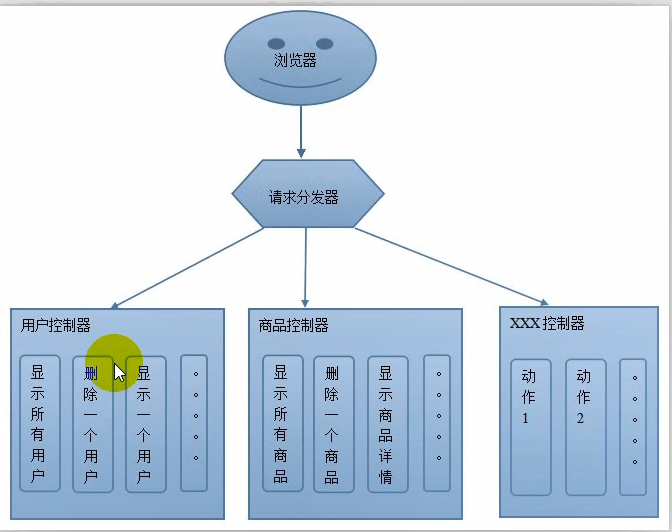index.php

``````require_once "./ModelFactory.class.php";
require_once "./BaseController.class.php";
\$ctrl = !empty(\$_GET['ctrl']) ? \$_GET['ctrl'] : "User";

require_once "./{\$ctrl}Model.class.php";

require+once "./{\$ctrl}Controller.class.php";

\$ctrl_name = \$ctrl."Controller";
\$controller = new \$ctrl_name();
\$action = !empty(\$_GET['action']) ? \$_GET['action'] : "Index";

\$action = \$action."Action";

\$controller->\$action();
``````

``````require_once "./ModelFactory.class.php";
require_once "./BaseController.class.php";
\$ctrl = !empty(\$_GET['ctrl']) ? \$_GET['ctrl'] : "User";

require_once "./{\$ctrl}Model.class.php";

require+once "./{\$ctrl}Controller.class.php";

\$controller = new \$ctrl_name();
\$action = !empty(\$_GET['action']) ? \$_GET['action'] : "Index";

\$action = \$action."Action";

\$controller->\$action();
``````

### 2.目录结构的设定

``````/Models
/Controllers
/Views
/Bases
index.php
``````

## 九、PDO 数据对象

### 1.概念：

PDO 是已经写好的数据库工具类，通过他能对数据库进行各种操作，非常的方便快捷

### 2.特点：

(1)他能操纵各种的数据库 ,mysql oracle sybase…
(2)他能实现除了增删改查以外的更多功能，比如事务
(3)他对不同的数据库有着统一的应用方式，在使用的时候无需考虑不同数据库SQL语法的细节差异
(4)PDO 实际上是一个类，我们使用的时候就需要new 一个对象出来

### 3.PHP 代码中常见的操作数据库的情况

(1)连接数据库得到数据库的连接资源
(2)执行各种sql 语句得到布尔值或者结果集
(3)对结果集的数据进行取用，遍历 一般就是 fetch

### 4.PDO 系统的逻辑结构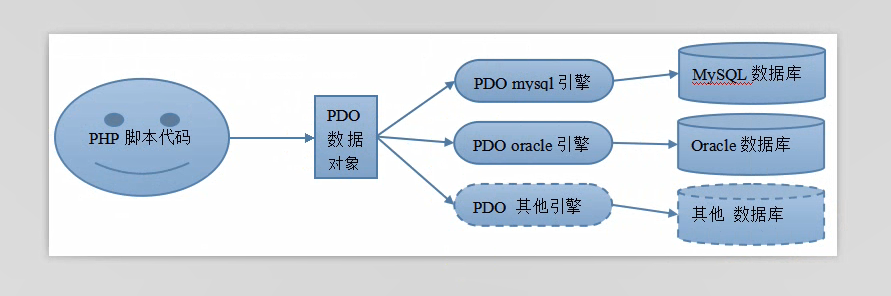### 5.POD 使用

#### 1.连接数据库

``````\$DSN = "mysql: host=服务器地址；port=端口号；dbname=数据库名"；
\$Options = array(PDO::MYSQL_ATTR_INIT_COMMADN => 'set names utf8');
\$pdo = new pdo(\$DNS,用户名,密码,\$Options);
``````

DSN : Data Source Name

#### 2.执行SQL语句

``````\$result1 = \$pdo->exec(增删改语句)；
\$result2 = \$pdo->query(各种sql语句)；
``````

exec 习惯上执行没有返回结果集的语句，返回值为 受影响的行数，当然也可能返回false

query 执行select 这种有返回结果集的语句，执行失败返回false,成功的话返回的结果集还需要进一步的处理。

#### 3.断开连接

``````\$pdo = null;
``````

### 6.POD 的错误处理

PDO 的错误处理有两种模式：

(1)静默模式:

``````\$pdo->exec(\$sql);
\$code = \$pdo->errorCode();//获取上一行代码执行的错误，如果没有错误则返回0、
if(\$code == 0){
echo "执行成功";
}
else{
\$info = \$pdo->errorInfo();
echo "失败,错误代号：".\$info;
}
``````

(2)异常模式:

``````try{
\$pdo->exec(\$sql);
}
catch(Exception \$e){
echo "发生错误，请参考错误提示：".\$e->GetMessage();
}
``````

### 7.POD 的结果集对象

#### 1.得到结果集对象：

``````\$res = \$pdo->query("select ...");
``````

#### 2.常见处理方法

``````\$res->rowCount();
``````

``````\$res->columnCount();
``````

``````\$res->fetch(返回类型)
``````

PDO::FETCH_ASSOC 返回关联数组
PDO::FETCH_NUM 返回索引数组
PDO::FETCH_BOTH 返回前两者皆有的数据（默认值）、
PDO::FETCH_OGJ 返回对象

``````\$res->fetchAll(返回类型);
``````

``````\$resds->fetchColumn([\$i]);
``````

### 8.POD 结果集对象的预处理语法、

#### 2.形式：

``````\$sql = "select * from user_list where age = ?";
``````

``````\$arr = array(11,28,32);
\$sql = "select * from user_list where age = ?";
\$result = \$pdo->prepare(\$sql);
foreach(\$arr as \$value){
\$result->bindVaule(1,\$value);//如果有多个问号就可以有多个语句
\$result->execute();
\$result = \$rersult->fetch(PDO::FETCH_ASSOC);
print_r(\$result);
}
``````

``````\$sql = "select * from user_list where age= :v1";
``````

``````\$sql = "select * from user_list where age = :v1 and edu = :v2";
\$result = \$pdo->prepare(\$sql);
\$result->bindVaule(":v1",11);
\$result->bindVaule(":v2","高中");
\$result->execute();
\$result = \$rersult->fetch(PDO::FETCH_ASSOC);
print_r(\$result);
``````